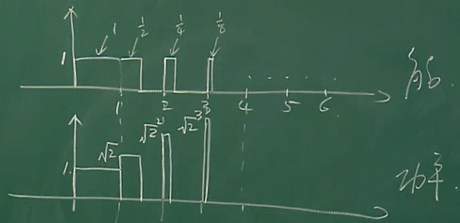# 第一章习题

1. 两个周期信号相加依然是周期信号？
2. 非周期信号一定是能量信号？
3. 能量信号一定是非周期信号？
4. 两个功率信号相加依然是功率信号？
5. 两个功率信号之积依然是功率信号？
6. 能量信号乘功率信号之积是能量信号？
7. 随机信号一定是非周期信号？

1. 两个周期信号相加依然是周期信号（×）
设周期信号 $x_1(t)=x_1(t+M)$，$x_2(t)=x_2(t+N)$
令 $x(t)=x_1(t)+x_2(t)$
要使 $x(t)=x(t+k)$，则要求 $k$ 是 $M,N$ 的（最小）公倍数。
即存在正整数 $P,Q$，使得 $k=QM=PN$，也就是要求：$\frac{M}{N}=\frac{P}{Q}$ 必须是有理数。显然当 $M,N$ 一个有理，一个无理时，相加后无周期。
更多讨论可以看 知乎：如果 f(x) 与 g(x) 均为周期函数，判断其相加后的周期性？

2. 非周期信号一定是能量信号（×）
考虑阶跃信号。

3. 能量信号一定是非周期信号（√）
周期信号能量一定无穷大，即一定是功率信号；而功率信号不可能是周期信号。所以周期信号一定不是能量信号，从而逆否命题：【能量信号一定是非周期信号】成立。（由于零信号无意义且无周期，我们不考虑零信号）。

4. 两个功率信号相加依然是功率信号（×)
一正一反相消。

5. 两个功率信号之积依然是功率信号（×）
一个是阶跃信号，一个是阶跃信号以y轴对称的信号，相乘为零。

6. 能量信号乘功率信号之积是能量信号（×）
emm……其实这个挺难想的。给出如下反例：能量信号：$x_1(t)=\sum_{k=0}^\infty [u(x-k)-u(x-\sum_{n=1}^k\frac{1}{2^n})]$ 级数收敛；
功率信号：$x_2(t)=\sum_{k=0}^\infty \sqrt{2}^k[u(x-k)-u(x-\sum_{n=1}^k\frac{1}{2^n})]$，级数发散；
相乘后依然是 $x_2(t)$，是功率信号。

7. 随机信号一定是非周期信号（×）
只要不能写出确定的函数式，则是随机信号。
比如：$\sin(x+H)$，其中，$H$ 是随机的。
显然上面的函数的周期为 $2\pi$，是周期信号，但我们无法画出这个信号。